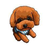V2EX = way to explore
V2EX 是一个关于分享和探索的地方

V2EX  ›  算法

# 关于余数，想求解是否有这样的关系

raysonlu · 115 天前 · 670 次点击

(axM)%N=b
(bxM)%N=a

22 条回复    2021-08-13 11:01:59 +08:001 aneostart173      115 天前 数论的东西，看看初等数论。2 raysonlu   OP   115 天前 @aneostart173 同余定理推算不出这个，或者您能给个初等数论中相关的理论？3 aneostart173      115 天前 @raysonlu 我不是数论的专家。。。这肯定是数论的知识，可以去数学论坛问啊。4 shoa      115 天前 via Android a × m mod n = bb × m mod n = a故 a × m × m mod n = a又因为 a ≠ 0m × m mod n = 1m = 1 或 m = n - 15 jie170601      115 天前 via Android 正好前端时间看了 RSA 算法，比这个复杂一点，这个引入个倍数 k 就好算一点了：aM=Nk1+bbM=Nk2+a把 M 消掉后可以得到 Nk=a^2+b^2，代回去也能得到 M 了，如果只是想得到一组 M 和 N 的话，不妨把倍数 k k1 k2 都取 1，严整的解析解没有去推，反正把求余符号转换成 nk+余数的形式就好计算很多了6 raysonlu   OP   115 天前 @jie170601 大哥，消 M 后得到的是 bNk1-aNk2 = a^2-b^2，你是怎样跳到“Nk=a^2+b^2”？在实际应用上，是得到了 M 和 N 这两个数字，然后 a 可以代入任何整数，实现 a 和 b 的关系转换。应该是有某种数学方法（不是通过 a 和 b)得到了 M 和 N 的7 jie170601      115 天前 不好意思，午休用手机敲的,看到方程好解就直接解了。ps：k 那个地方是令 k=bk1-ak2，下面的推导也会经常合并 k 。再尝试推一下：aM=Nk1+bbM=Nk2+a消去 a：(bM-Nk2)M = Nk1+b合并 N：N(k2M+k1)=b-bM^2即：Nk3=b-bM^2两边同除 b：M^2=1+Nk即：M^2 % N = 1根据欧拉定理 /欧拉公式：f(N)=2M 为与 N 互质的任意整数临时想到的，推导可能有错，用欧拉定理的方向应该没问题😂8 Quantumzhao      114 天前 不知道楼主现在问题解决了没有……不过或许可以有另一种思路首先可以把上面的等式重写为：（下面的操作都是在 Z/NZ 的群中）aM ≡ bbM ≡ a因为 Z/NZ 是一个环，所以移项（以及等式两边同时加减）可得：① (a + b)M ≡ (a + b)② (a - b)M ≡ (b - a)然后分类讨论。若 M∈ 那么根据 ② 式就有：b - a ≡ 0，即 a ≡ b代入 ① 式可得a + b ≡ 0因此 a ≡ b ≡ 0若 M∈ 那么根据 ② 式就有：a - b ≡ b - a，即 a ≡ b若 M∈[-1] 那么根据 ① 式就有：a + b ≡ -(a + b)，即 a + b ≡ 0若 M∈Z/NZ -  -  - [-1]，那么根据 ① 式就有：a + b ≡ 0，即 a ≡ -b将其带入 ② 中，可得(-b - b)M ≡ 2b-2bM ≡ 2b2b(M + 1) ≡ 0因为 M 不在 [-1] 中，所以 2b ≡ 0总结：- 如果 M 是 N 的倍数，那么 a 和 b 都是 N 的倍数- 如果 M = kN + 1，k 为任意整数，那么 a 和 b 的余数相同- 如果 M = kN - 1，k 为任意整数，那么 a + b 是 N 的倍数- 如果 M 是其他情况，那么 a + b 是 N 的倍数且 2b 是 N 的倍数可能还有些错误和不完整的地方9 ziwiwiz      114 天前 M = a+b-1N = a+b验证，存在整数 x,y 满足aM=xN+bbM=yN+a展开a(a+b-1)=x(a+b)-bb(a+b-1)=y(a+b)-ax=(aa+ab-a-b)/(a+b)=a-1y=(bb+ab-a-b)/(a+b)=b-110 ziwiwiz      114 天前 @ziwiwiz #9 M = a+b-1N = a+b验证，存在整数 x,y 满足aM=xN+bbM=yN+a代入展开a(a+b-1)=x(a+b)+bb(a+b-1)=y(a+b)+ax=(aa+ab-a-b)/(a+b)=a-1y=(bb+ab-a-b)/(a+b)=b-1举例 a=232 b=678M=909 N=910 x=231 y=677(232*909)%910=678(678*909)%910=23211 raysonlu   OP   114 天前 @jie170601 感觉上，方向貌似有点正确，不过不是很理解，在“合并 N”步骤中，不是应该得到“N(k2M+k1) = -b-bM^2”吗？接下来的，把“(k2M+k1)”换成了 k3，是什么原理？（里面有 M 哦）如果修正了“合并 N”步骤那里，并强行理解 k3，那最后就是“-(M^2) % N =1”。不过我尝试了游戏啊，根据你的结果得到一个 M 和 N 再用来演算式子，貌似走得通。（所以结论是任意一个互质的整数可以符合题目的关系？）12 raysonlu   OP   114 天前 @Quantumzhao 开头的重写，小弟我就看不懂了，那个“等号”是指恒等于还是同余？不过后面的方向，貌似与题意也不符合，其实题意希望是：找出一组（或多组）的 M 和 N，代入任意正整数 a，a13 raysonlu   OP   114 天前 @ziwiwiz 你的这个假设是成立的，但不知道是怎么假设出来的，以及貌似不是完全解。比如按照暂时#7 的结论是正确的话，即 M^2 % N = 1，那么可以得到一个 M=66，N=871，然后可以代入一个 a=123，得出一个 b=279，并且也符合原题式子。但这个结果不属于这个假设。14 Quantumzhao      114 天前 via Android @raysonlu 对，就是同余的意思。其实最终的结论我是想说，M 和 N 可以取任意值（总是存在一对满足这样条件的 M 和 N ），然后如果还想另外知道此时 a 和 b 的关系的话，也可以参考“如果...” 的后半段(其实说白了基本上是其他几楼答案的汇总,不过更加 general 一些15 raysonlu   OP   114 天前 @Quantumzhao 同余是与哪个模同余？ aM 和 b 不是模 N 的同余吧？16 jie170601      114 天前 @raysonlu 结论并不是任意一个互质的整数可以符合题目的关系还有个 f(N)=2 的前提不过我那样求解实用性不大，M 和 N 需要满足 M^2 % N = 1，然后欧拉定理刚好可以保证 M 和 N 互质时 M^f(N) % N = 1,只要 f(N)等于 2 就行了，f 表示欧拉函数，但是这时候 N 就只能取 3，4，6，M 倒是可以任意取，只要与 N 互质隐含条件 a17 raysonlu   OP   114 天前 @jie170601 #16 的“然后欧拉定理。。。”后面我看得不是很明白（毕竟很少研究算法云云），不过我刚开始的时候，直接拿“ M^2 % N = 1”作为结论去生成各种不同的 M 和 N，的确能满足题目的关系，然后回头演算了一下你给出的推导过程（然后我在上一个回复发现了一个推导出现了一个错误是不是我理解不正确？）anyway，可以 TG 一下再进一步沟通？ QFJheXNvbkx118 jie170601      114 天前 via Android @raysonlu 好像是有两步符号都算反了导致结论没出问题，欧拉定理可以搜一下，比较好看懂的。如果推导过程没错，只要满足 M^2 % N = 1 的 M 和 N 都是符合原题式子的，至于后面互质、欧拉定理那些只是求解这类 M，N 的一种方法19 Quantumzhao      113 天前 @raysonlu 我想说的是 aM congruent to b比如第一个等式的意思是 aM = kN + b，k 为任意整数且 a 和 b < N 且 >= 0 。因此 aM 除以 N 的余数就是 b 。而 b 的余数也是 b，所以 aM 和 b 是模 N 的同余（我应该没有理解错同余的意思……？）然后因为我接下来都是用整数模 N 的群的性质，所以就省略了 N20 raysonlu   OP   113 天前 @jie170601 其实我也是见别人用这种方法，进行 a 和 b 的转换，其实在后面，我更想了解这种方法，是怎么被发掘出来的？我还以为这已经是一个数学定论21 raysonlu   OP   113 天前 @Quantumzhao “因此 aM 除以 N 的余数就是 b”这个从题目中明显得到，但“而 b 的余数也是 b”这里我还是推敲不出来，b 与 N 取模怎样等于 b 了？大佬在这里跳快了小弟跟不上。同余的意思就是教科书般简单没问题。22 Quantumzhao      113 天前 @raysonlu 比如说 5 mod 6，那么 5 可以写成 0 × 6 + 5 。然后根据取模的约定俗成的习惯，因为加号后面的那个 5 < 6 且 ≥ 0，所以 5 的余数就是 5 。同样如果把 5 和 6 替换成其他任意数字（比如 b 和 N ）也是一样的（因为 b < N 且 ≥ 0 ）另外后面的证明里我用到了和某个负数同余，例如 a ≡ -b，其实意思就是 a 和 N - b 同余